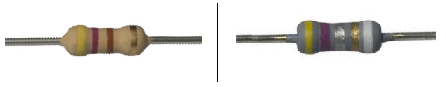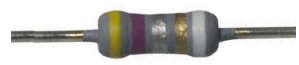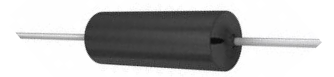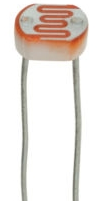﻿ Types of ResistorsTypes of ResistorsThere are many different types of resistors and they each vary in their composition, characteristics, and functions.

Some resistors are composed of carbon. Others are composed of metal-film. Some resistors are used as light sensors. Others are used to measure heat.

We will first go over the different types of resistors based on their composition, what materials they are internally composed of. Then we will go over all the different types of resistors classified by the functions they perform.

Types of Resistors by Composition

There are 3 main types of resistors based on their composition: carbon-composition resistors, carbon-film resistors, and metal-film resistors.

Carbon-composition ResistorsCarbon-composition resistors are resistors that are made of finely divided carbon or graphite mixed with a powdered insulating material as a binder in the proportions need for the desired R value.

More carbon produces less resistance, while more binder equals greater resistance. The resistor element is enclosed in a plastic case for insulation and mechanical strength. Joined to the two ends of the carbon resistance element are metal caps with leads of tinned copper wire for soldering the connections into a circuit. Carbon-composition resistors normally have a brown body and are cylindrical.

Carbon-composition resistors are commonly available in resistance values of 1Ω to 20MΩ. The power rating is generally 0.1, 0.125, 0.25, 0.5, 1, or 2W.

Carbon-film ResistorsA carbon-film resistor is a resistor in which a thin film of carbon is deposited onto an insulated substrate and then cut into a spiral body.

The resistance value of the resistor is controlled by varying the proportion of carbon to insulator. More carbon gives less resistance, while more insulating material gives greater resistance.

Compared to carbon-composition resistors, carbon-film resistors have the following advantages: lower and tighter tolerances, less sensitivity to temperature changes and aging, and less noise generated internally.

Metal-film ResistorsA metal-film resistor is a resistor in which a thin film of metal is sprayed onto a ceramic substrate and then cut into a spiral body.

The length, thickness, and width of the metal spiral determine the exact resistance value.

Metal-film resistors offer more precise resistance values than the other type of film resistors, which are carbon-film resistors. Like carbon-film resistors, metal-film resistors are affected very little by temperature changes and aging. They also generate very little noise internally.

Between the three types of resistors based on composition, carbon-composition resistors, carbon-film resistors, and metal-film resistors, in overall performance, metal-film resistors are the best, carbon-film the next best, and carbon-composition resistors last.

Types of Resistors by Function

Power Wirewound ResistorsPower wirewound resistors are resistors that can handle a large amount of power, usually up to 50 watts.

Typical resistors normally can handle between 0.25W to 2W of power. Power wirewound resistors can handle much, much more and are suitable for use in high-power applications.

Precision ResistorsPrecision Resistors are resistors that have very low tolerance values, so they are very precise (closely near their nominal value).

All resistors come with a tolerance value, which is given as a percentage. The tolerance value tells us how close to the nominal value a resistance can vary. For example, a 500Ω resistor that has a tolerance value of 10%, may have a resistance that is somewhere between 10% above 500Ω (550Ω) or 10% below 500Ω (450Ω). If the same resistor has a tolerance of 1%, its resistance will only vary by 1%. Therefore, a 500Ω resistor can then vary between 495Ω and 505Ω. This is tolerance as an example.

A precision resistor is a resistor that has a tolerance level as low as 0.005%.

This means a precision resistor will only vary 0.005% from its nominal value.

Precision resistors have very low tolerance percentage values, making them ultraprecise to their nominal values. They will vary very little from their nominal values, so they are used in applications where high-precision is necessary in terms of resistance values.

Fusible ResistorA Fusible Resistor is a wire-wound resistor that is designed to burn open easily when the power rating of the resistor is exceeded.

In this way, a fusible resistor serves dual functions. When the power isn't exceeded, it serves as a resistor limiting current. When the power rating is exceeded, it functions as a fuse, burning up, and becoming an open in the circuit to protect components in the circuit from excess current.

Cement ResistorsCement resistors are power resistors that are heat and flame resistant.

Cement resistors are made to handle a large amount of power flowing through it with it being undamaged by heat or flames. If you are designing a circuit where a lot of current is being passed through a resistor and with it needing to be resistant to high heat and flame, cement resistors is a good design choice.

Typical power ratings range from 1W to 20W or more.

Tolerances from the stated resistance value are around 5 percent.

PotentiometersA potentiometer is a 3-terminal variable resistor. By adjusting the wiper terminal, it can be used to represent a wide range of resistances in a circuit from anywhere near 0Ω to the specified resistance rating of the potentiometer. Therefore, for example, a 10KΩ potentiometer can be adjusted to give the resistance range from almost 0Ω to 10KΩ by adjusting the potentiometer knob.

Potentiometers are in wide use in circuits for a variety of uses, but their main fuction remains the same: to increase or decrease the amplitude of a signal in a circuit. When the resistance of the potentiometer is decreased, the amplitude of the signal increases. When the resistance is increased, the amplitude of the signal decreases. This can be used in circuits to control volume levels such as on speakers or for any adjustable controls.

RheostatsA rheostat is a 2-terminal variable resistor.

As a variable resistor, it serves to vary the amount of voltage or current in a circuit.

Just like potentiometers, rheostats can be used to vary AC or DC signals. They differ from potentiometers in the fact that they only have two leads of which potentiometers have 3. However, the two still achieve the same function. While a potentiometer uses a third terminal to serve as the adjustable part, the rheostat uses a slider to vary resistance. If the slider isn't moved, the rheostat functions as a fixed resistor.

A rheostat is connected in a circuit by having the two leads connected in series with the load.

ThermistorsA thermistor is a thermally sensitive resistor whose resistance value changes with changes in operating temperature.

Because of the self-heating effect of current in a thermistor, the device changes resistance with changes in current.

Thermistors exhibit either a positive temperature coefficient (PTC) or a negative temperature coefficient (NTC). If a thermistor has a positive temperature coefficient, its resistance increases as the operating temperature increases. Conversely, if a thermistor has a negative temperature coefficient, its resistance decreases as the operating temperature increases.

How much the resistance changes with changes in the operating temperature depends on the size and construction of the thermistor. It's always best to check the datasheet of the thermistor in use to find out all the specifications of the thermistors.

Thermistors are frequently used in electronic circuits that handle temperature measurement, temperature control, and temperature compensation.

PhotoresistorsPhotoresistors are resistors whose resistance values change according to the light striking the surface of the resistor. In a dark environment, the resistance of a photoresistor is very high, possibly several MΩ, depending on the resistance rating of the specific photoresistor in use. When intense light hits the surface, the resistance of the photoresistor drops dramatically, possibly to as low as 400Ω.

Thus, photoresistors are variable resistors whose resistance values change in regard to the amount of light hitting its surface.

The above list is an extensive list of the types of resistors which exist today. The last 4 resistors are variable resistors which change according to knob adjustments, heat, and light, respectively.

Related Resources